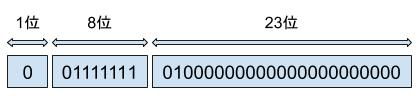### 为什么浮点精度运算会有问题

``````double num = 0.1 + 0.1 + 0.1;
// 输出结果为 0.30000000000000004
double num2 = 0.65 - 0.6;
// 输出结果为 0.05000000000000004``````

``````// 二进制到十进制
10010 = 0 * 2^0 + 1 * 2^1 + 0 * 2^2 + 0 * 2^3 + 1 * 2^4 = 18

// 十进制到二进制
18 / 2 = 9 .... 0
9 / 2 = 4 .... 1
4 / 2 = 2 .... 0
2 / 2 = 1 .... 0
1 / 2 = 0 .... 1

10010``````

``````// 二进制到十进制
10.01 = 1 * 2^-2 + 0 * 2^-1 + 0 * 2^0 + 1 * 2^1 = 2.25

// 十进制到二进制
// 整数部分
2 / 2 = 1 .... 0
1 / 2 = 0 .... 1
// 小数部分
0.25 * 2 = 0.5 .... 0
0.5 * 2 = 1 .... 0

// 结果 10.01``````

``````2.1 分成两部分
// 整数部分
2 / 2 = 1 .... 0
1 / 2 = 0 .... 1

// 小数部分
0.1 * 2 = 0.2 .... 0
0.2 * 2 = 0.4 .... 0
0.4 * 2 = 0.8 .... 0
0.8 * 2 = 1.6 .... 1
0.6 * 2 = 1.2 .... 1
0.2 * 2 = 0.4 .... 0
0.4 * 2 = 0.8 .... 0
0.8 * 2 = 1.6 .... 1
0.6 * 2 = 1.2 .... 1
0.2 * 2 = 0.4 .... 0
0.4 * 2 = 0.8 .... 0
0.8 * 2 = 1.6 .... 1
0.6 * 2 = 1.2 .... 1
............``````

### 精度运算丢失的解决办法

1. 如果业务不是必须非常精确的要求可以采取四舍五入的方法来忽略这个问题。
2. 转成整型再进行计算。
3. 使用 BCD 码存储和运算二进制小数(感兴趣的同学可自行搜索学习)。

``````# Python 示例
from decimal import Decimal

num = Decimal('0.1') + Decimal('0.1') + Decimal('0.1')
print(num)``````
``````// Java 示例
import java.math.BigDecimal;

### 拓展：详解浮点型0 是正数，1 是负数

##### 尾数位

``````import struct

num = 1.1
bins = ''.join('{:0>8b}'.format(c) for c in struct.pack('!f', num))
print(bins)``````
Java 版本的代码有点长，我就放个链接了。
Java 版代码链接